# ISEE Middle Level Math : How to find median

## Example Questions

### Example Question #71 : How To Find Median

Find the median of this set of numbers: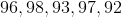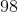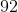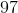Explanation:

First order the numbers from least to greatest: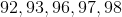Then identify the middle number:Answer:### Example Question #71 : Median

Find the median of this set of numbers: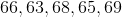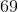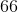Explanation:

First, order the numbers from least to greatest: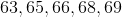Then, identify the middle number:Answer:### Example Question #73 : How To Find Median

Find the median in this set of numbers: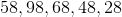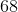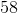Explanation:

First order the numbers from least to greatest: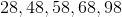Identify the middle number:Answer:### Example Question #74 : How To Find Median

Find the median of this set of numbers: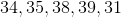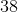Explanation:

First order the numbers from least to greatest: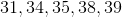Then identify the middle number:Answer:### Example Question #71 : How To Find Median

What is the median of the set below?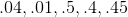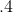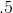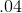Explanation:

The first step to finding the median is to rearrange the numbers in order from smallest to largest.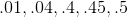The median is the middle number of a set when the numbers of a set are ordered from smallest to largest.

The middle number of the set above, ordered from smallest to largest, is .4. Therefore, .4 is the correct answer.

### Example Question #71 : How To Find Median

Shelly took five tests and quizzes this semester in school. If her grades were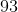,,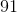,, and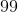, what is her median test score?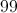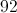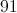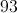Explanation:

First, order the test scores from least to greatest: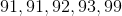Identify the middle test score:Answer: Shelley's median test score is 92.

### Example Question #77 : How To Find Median

Find the median of the following set of numbers: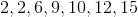Explanation:

To find the median, simply find the number in the middle, with equal quantities of numbers on either side after your list has been ordered from smallest to greatest.

Our dataset is already in ascending order and we have a total of seven entires. Therefore the middle of the set will lie where there are three entries to the left and three entries to the right.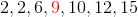### Example Question #71 : Median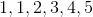How would the median of the above set change if theis replaced by?

It increases

It decreases

Not enough information to find solution

It stays the same

It stays the same

Explanation:

The median is the number that falls in the middle of a set when ordered by smallest to largest.  The median is decided by placement among the other values and not a calculation.  Since only the highest value is changed, the middle number will remain the same value.

### Example Question #72 : How To Find Median

Find the median of the following data set: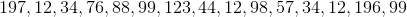Explanation:

Find the median of the following data set:First, let's rearrange our numbers in increasing order: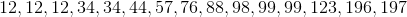Now, the median will simply be the term which is in the middle of our data set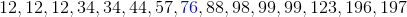### Example Question #73 : How To Find Median

Find the median of the following data set: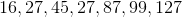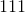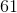Explanation:

Find the median of the following data set: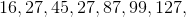First, let's put our set in increasing order: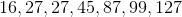Next, identify the median by identifying the term in the middle of our data set:In this case, 45 is our median

### All ISEE Middle Level Math Resources